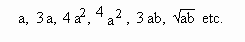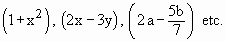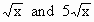Home MonkeyNotes Printable Notes Digital Library Study Guides Study Smart Parents Tips College Planning Test Prep Fun Zone Help / FAQ How to Cite New Title Request

 3.2 Monomials, Polynomials and Factoring Monomial : An algebraic expression which contains only one term is called the monomial expression. For exampleBinomial : An algebraic expression consisting of two terms is called a Binomial expression. For example, ( 4 a + 5 b ),Similarly, we can define trinomial etc. Like terms : Terms which involve the same letter and the same index ( i.e. power ) are ‘like terms’ . For example 2a and 3 a ,,, 3 xy and - xy etc. Addition and Subtraction of Monomials : To add or subtract monomials, use the same rules as with directed (signed) numbers, provided the terms are alike. Example Add 20 a2 b c + 4 a2 b c Solution : 20 a2 b c + 4 a2 b c = ( 20 + 4 ) a2 b c                                                = 24 a2 b c Note that the addition of like terms is done by adding their coefficients (taken with their signs ) and multiplying this sum by the common letter or letters. Perform the operations indicated : Example 7 x - 2 x Solution : 7 x - 2 x = ( 7 - 2 ) x                                = 5 x Example 10 x2 + 3 x2 - 5 x2 Solution : 10 x2 + 3 x2 - 5 x2 = ( 10 + 3 - 5 ) x2                                                = 8 x2 Index 3.1 - Introduction3.2 - Monomial, Polynomials3.3 - H.C.F and L.C.M Chapter 4
All Contents Copyright © All rights reserved.
Further Distribution Is Strictly Prohibited.

 Search: All Products Books Popular Music Classical Music Video DVD Toys & Games Electronics Software Tools & Hardware Outdoor Living Kitchen & Housewares Camera & Photo Cell Phones Keywords: